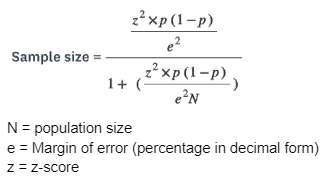# Sample Size Calculator

Confidence Level (%)

0.00 Sample Size

The sample size is a special term for the number of subjects who take part in an experiment, study, or survey. It is quite difficult to interview a large audience (for example, the city population). Therefore, a definite segment of the population receives questionnaires. If you want to know what kind of special nutrition arm wrestlers prefer, then it is unrealistic to get the opinion of all sportsmen (to organize a survey so that everyone is a participant). But it is possible to take at least 1000 individuals and analyze their views. Required sample size calculator considers the confidence interval (or margin of error). This allows analysis to base on a positive or negative number in a particular study.

## How to Calculate Sample Size?

The statistical sample size calculator is a significant platform saver because research requires a lot of money, time, and energy. At the same time, any test or experiment (human sources surveys, market research, customer satisfaction studies, and so on) should start with collecting specific knowledge. Before you'll use a calculator and get the result, you need to know the following info:

• sample size calculations based on hypotheses (minimum from 3);
• prove that your test or research is considerable;
• know the scientific interest of your analysis;
• find out all parameters that are necessary to obtain the result in calculations with formula;
• designate the test power (0.8 is min).

For data analysis, a statistician or any other person who conducts research must perform a calculation using a formula. If you have completed these points, it is not hard to compute the data using it. It is important to remember that the more accurate the parameters, the more exact the results are. A perfect choice is to do the calculation online with a Raosoft sample size calculator. Strive for maximum indicator - the test power for obtaining a grant is ideally 0.9.

## Determining Sample Size

The collecting information process and calculating the formula has a gap. Explore a few useful factors that determine your research. These steps allow you to obtain a basic understanding of the statistics involved. Note that such stages determine the sample size only for continuous info, not categorical data (red, yellow, female, male, and others).

1. Formula variables (people quantity, standard deviation, margin of error, confidence level).
2. Calculation with paper or sample size needed calculator (confidence level is a Z-score).
3. Use sample size formula - (Z-score)2 multiplied by StdDev and (1-StdDev), divide this by (margin of error)2.p = population proportion

To meet any survey aim, you should increase the list of studied participants. Multiple survey channels will allow you to reach more personalities (surveys on websites, SMS, etc.). All participants must receive test results after calculation and analysis. And don't forget to add motivation for encouraging people (bonuses, prize draws).

The virtual calculator is excellent because it is easier and more convenient for more exact analysis. You define your parameters and paste them into the appropriate fields for the calculator to answer.

## Optimal Sample Size

If a circle of respondents is too huge, it is somewhat troublesome, time-consuming, and costly to interview everyone. To organize a survey, there is a sample, that is, the selection of a certain personality number that best fits a definite test. A good solution is a random selection of a group of persons where everyone from numerous participants surveyed could be suitable.

Sample size calculator proportion based on a specific formula; as a result, you get parameters from 200 people and not from 20,000. If you study the opinion of a citizen where there are 500,000 inhabitants, then it would be less resource-saving to study the specific position of 1000 of them.

If 40% of participants in the reduced crew answer positively, then the margin of error is 2 percent. This means that researching the entire people team, the probability of their answer is range from 38 to 42%. The optimal sample size is a position above 30 percent. This is a normal distribution. The smaller indicator (Student's t-distribution) supports by sample size calculators with standard deviation.

## Conclusion

To get personalities' opinions on a particular topic, you need a sample size calculator. Research to append certain parameters: the main ones are confidence interval, a margin of error, and confidence level. After identifying this data, you receive the result using a formula and Internet calculator.

Articles from the blog